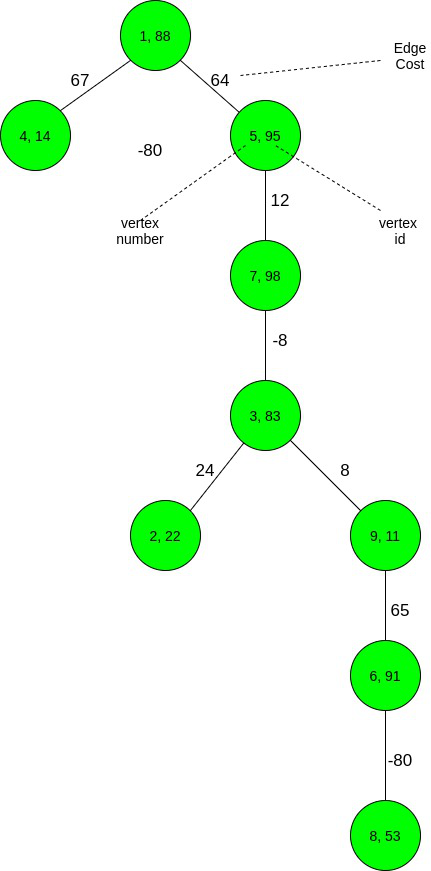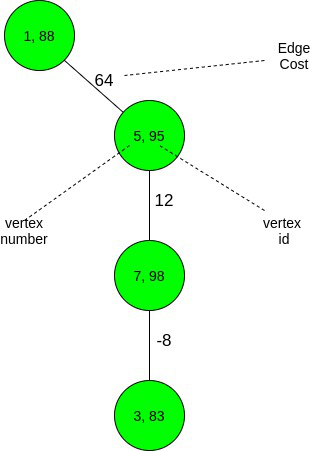Related Articles

# Minimum number of leaves required to be removed from a Tree to satisfy the given condition

• Difficulty Level : Hard
• Last Updated : 01 Sep, 2021

Given a Tree consisting of N vertices, rooted at vertex 1 and an array val[] representing the values assigned to each vertex, and an array cost[] representing the cost of each edge in the Tree, the task is to find the minimum number of leaves to be removed from the given tree such that:

For any vertex V, the sum of cost of edges to a vertex U in its subtree never exceeds val[U].

Examples :

Input: N = 9, val[] = {88, 22, 83, 14, 95, 91, 98, 53, 11},
cost[] = { -1, 24, -8, 67, 64, 65, 12, -80, 8 }Output:
Explanation:
The final graph after necessary removal of leaves is as follows:Cost of edges (1, 4) = 67 > 14 (= val). Therefore vertex 4 is removed.
Cost of edges (3, 2) = 24 > 22 (= val). Therefore, vertex 2 is removed.
Cost of edges (1 –> 5 –> 7 –> 3 –> 9) = 64 + 12 + 8 – 8 = 76 > 11 (= val). Therefore, vertex 9 needs to be deleted. Therefore, the entire subtree {9, 6, 8} is deleted.
Therefore, 5 nodes are removed from the tree.

Input: N = 7, val[] = { 11, 16, 27, 21, 15, 9, 11 },
cost[] = { 12, 1, 7, -17, -2, 2, 17}
edges[] = {{0, 3}, {0, 4}, {3, 6}, {4, 2}, {2, 1}, {2, 5}}
Output: 7

Approach:
Follow the steps below to solve the problem:

• If for a vertex V, there is a vertex U such that edge cost (V –> U) exceeds val[U], there is no other choice except to delete the entire subtree rooted at U or at V.This is because we are only allowed to delete the leaf vertices.
• Since only leaf vertex can be deleted, to delete U or V, the whole subtree needs to be deleted leaf by leaf in order to reach the vertex U or V.
• To minimize the number of leaves to be deleted, greedily select the subtree with a lesser number of vertices, i.e. V’s subtree will be deleted if the number of vertices in V‘s subtree exceeds the number of vertices in U‘s subtree, and vice versa.
• Apply Depth First Search on the tree and for every unvisited vertex, check whether if it satisfies the required condition.
• Increase count for every vertex satisfying the condition. For vertices not satisfying the condition, no need to traverse its subtrees as it is needed to delete entirely.
• Finally, print N – count, after complete traversal of the tree as count contains the number of vertices that are not required to be deleted.

Below is the Implementation of the above-explained approach:

## C++

 `// C++ Program to find the minimum``// number of leaves to be deleted``#include ``using` `namespace` `std;`` ` `// Stores the count of safe nodes``int` `cnt = 0;`` ` `// Function to perform DFS on the Tree``// to obtain the count of vertices that``// are not required to be deleted``void` `dfs(``int``* val, ``int``* cost,``         ``vector >& tr,``         ``int` `u, ``int` `s)``{``    ``// Update cost to reach``    ``// the vertex``    ``s = s + cost[u];``    ``if` `(s < 0)``        ``s = 0;`` ` `    ``// If the vertex does not``    ``// satisfy the condition``    ``if` `(s > val[u])``        ``return``;`` ` `    ``// Otherwise``    ``cnt++;`` ` `    ``// Traverse its subtree``    ``for` `(``int` `i = 0; i < tr[u].size(); i++) {``        ``dfs(val, cost, tr, tr[u][i], s);``    ``}``}`` ` `// Driver Code``int` `main()``{``    ``int` `n = 9;``    ``int` `val[] = { 88, 22, 83, 14, 95,``                  ``91, 98, 53, 11 };``    ``int` `cost[] = { -1, 24, -8, 67, 64,``                   ``65, 12, -80, 8 };`` ` `    ``// Stores the Tree``    ``vector > tr(n + 1);``    ``tr.push_back(3);``    ``tr.push_back(4);``    ``tr.push_back(6);``    ``tr.push_back(2);``    ``tr.push_back(1);``    ``tr.push_back(8);``    ``tr.push_back(5);``    ``tr.push_back(7);`` ` `    ``// Perform DFS``    ``dfs(val, cost, tr, 0, 0);`` ` `    ``// Print the number of nodes``    ``// to be deleted``    ``cout << n - cnt;`` ` `    ``return` `0;``}`

## Java

 `// Java Program to find the minimum``// number of leaves to be deleted``import` `java.util.*;``class` `GFG{`` ` `// Stores the count of safe nodes``static` `int` `cnt = ``0``;`` ` `// Function to perform DFS on the Tree``// to obtain the count of vertices that``// are not required to be deleted``static` `void` `dfs(``int` `[]val, ``int` `[]cost,``                ``Vector []tr,``                ``int` `u, ``int` `s)``{``  ``// Update cost to reach``  ``// the vertex``  ``s = s + cost[u];``  ``if` `(s < ``0``)``    ``s = ``0``;`` ` `  ``// If the vertex does not``  ``// satisfy the condition``  ``if` `(s > val[u])``    ``return``;`` ` `  ``// Otherwise``  ``cnt++;`` ` `  ``// Traverse its subtree``  ``for` `(``int` `i = ``0``; i < tr[u].size(); i++) ``  ``{``    ``dfs(val, cost, tr, tr[u].get(i), s);``  ``}``}`` ` `// Driver Code``public` `static` `void` `main(String[] args)``{``  ``int` `n = ``9``;``  ``int` `val[] = {``88``, ``22``, ``83``, ``14``, ``95``,``               ``91``, ``98``, ``53``, ``11``};``  ``int` `cost[] = {-``1``, ``24``, -``8``, ``67``, ``64``,``                ``65``, ``12``, -``80``, ``8``};`` ` `  ``// Stores the Tree``  ``@SuppressWarnings``(``"unchecked"``)``  ``Vector []tr = ``new` `Vector[n + ``1``];``   ` `  ``for` `(``int` `i = ``0``; i < tr.length; i++)``    ``tr[i] = ``new` `Vector();``  ``tr[``0``].add(``3``);``  ``tr[``0``].add(``4``);``  ``tr[``4``].add(``6``);``  ``tr[``6``].add(``2``);``  ``tr[``2``].add(``1``);``  ``tr[``2``].add(``8``);``  ``tr[``8``].add(``5``);``  ``tr[``5``].add(``7``);`` ` `  ``// Perform DFS``  ``dfs(val, cost, tr, ``0``, ``0``);`` ` `  ``// Print the number of nodes``  ``// to be deleted``  ``System.out.print(n - cnt);``}``}`` ` `// This code is contributed by Princi Singh`

## Python3

 `# Python3 program to find the minimum``# number of leaves to be deleted`` ` `# Stores the count of safe nodes``cnt ``=` `0`` ` `# Function to perform DFS on the Tree``# to obtain the count of vertices that``# are not required to be deleted``def` `dfs(val, cost, tr, u, s):``     ` `    ``global` `cnt``     ` `    ``# Update cost to reach``    ``# the vertex``    ``s ``=` `s ``+` `cost[u]``     ` `    ``if` `(s < ``0``):``        ``s ``=` `0`` ` `    ``# If the vertex does not``    ``# satisfy the condition``    ``if` `(s > val[u]):``        ``return`` ` `    ``# Otherwise``    ``cnt ``+``=` `1`` ` `    ``# Traverse its subtree``    ``for` `i ``in` `range``(``0``, ``len``(tr[u])):``        ``dfs(val, cost, tr, tr[u][i], s)``     ` `# Driver Code``if` `__name__ ``=``=` `"__main__"``:``     ` `    ``n ``=` `9``    ``val ``=` `[ ``88``, ``22``, ``83``, ``14``, ``95``, ``            ``91``, ``98``, ``53``, ``11` `]``    ``cost ``=` `[ ``-``1``, ``24``, ``-``8``, ``67``, ``64``, ``             ``65``, ``12``, ``-``80``, ``8``]`` ` `    ``# Stores the Tree``    ``tr ``=` `[[] ``for` `i ``in` `range``(n ``+` `1``)]``    ``tr[``0``].append(``3``)``    ``tr[``0``].append(``4``)``    ``tr[``4``].append(``6``)``    ``tr[``6``].append(``2``)``    ``tr[``2``].append(``1``)``    ``tr[``2``].append(``8``)``    ``tr[``8``].append(``5``)``    ``tr[``5``].append(``7``)`` ` `    ``# Perform DFS``    ``dfs(val, cost, tr, ``0``, ``0``)`` ` `    ``# Print the number of nodes``    ``# to be deleted``    ``print``(n ``-` `cnt)``     ` `# This code is contributed by rutvik_56`

## C#

 `// C# Program to find the minimum``// number of leaves to be deleted``using` `System;``using` `System.Collections.Generic;``class` `GFG{`` ` `// Stores the count of safe nodes``static` `int` `cnt = 0;`` ` `// Function to perform DFS on the Tree``// to obtain the count of vertices that``// are not required to be deleted``static` `void` `dfs(``int` `[]val, ``int` `[]cost,``                ``List<``int``> []tr,``                ``int` `u, ``int` `s)``{``  ``// Update cost to reach``  ``// the vertex``  ``s = s + cost[u];``  ``if` `(s < 0)``    ``s = 0;`` ` `  ``// If the vertex does not``  ``// satisfy the condition``  ``if` `(s > val[u])``    ``return``;`` ` `  ``// Otherwise``  ``cnt++;`` ` `  ``// Traverse its subtree``  ``for` `(``int` `i = 0; i < tr[u].Count; i++) ``  ``{``    ``dfs(val, cost, tr, tr[u][i], s);``  ``}``}`` ` `// Driver Code``public` `static` `void` `Main(String[] args)``{``  ``int` `n = 9;``  ``int` `[]val = {88, 22, 83, 14, 95,``               ``91, 98, 53, 11};``  ``int` `[]cost = {-1, 24, -8, 67, 64,``                ``65, 12, -80, 8};`` ` `  ``// Stores the Tree  ``  ``List<``int``> []tr = ``new` `List<``int``>[n + 1];``   ` `  ``for` `(``int` `i = 0; i < tr.Length; i++)``    ``tr[i] = ``new` `List<``int``>();``   ` `  ``tr.Add(3);``  ``tr.Add(4);``  ``tr.Add(6);``  ``tr.Add(2);``  ``tr.Add(1);``  ``tr.Add(8);``  ``tr.Add(5);``  ``tr.Add(7);`` ` `  ``// Perform DFS``  ``dfs(val, cost, tr, 0, 0);`` ` `  ``// Print the number of nodes``  ``// to be deleted``  ``Console.Write(n - cnt);``}``}`` ` `// This code is contributed by Rajput-Ji`

## Javascript

 ``
Output:
`5`

Time Complexity: O(N)
Auxiliary Space: O(N)

Attention reader! Don’t stop learning now. Get hold of all the important DSA concepts with the DSA Self Paced Course at a student-friendly price and become industry ready.  To complete your preparation from learning a language to DS Algo and many more,  please refer Complete Interview Preparation Course.

In case you wish to attend live classes with experts, please refer DSA Live Classes for Working Professionals and Competitive Programming Live for Students.

My Personal Notes arrow_drop_up